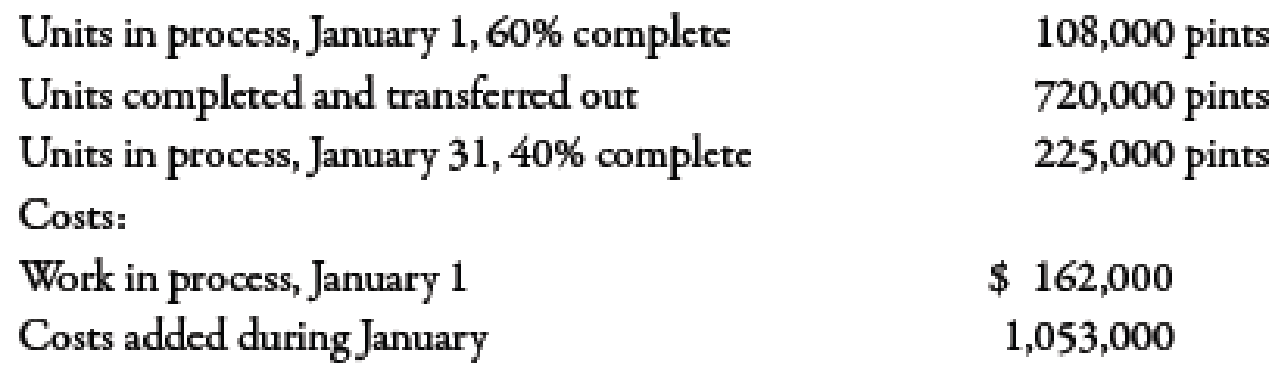Chapter 6, Problem 24BEA### Managerial Accounting: The Corners...

7th Edition
Maryanne M. Mowen + 2 others
ISBN: 9781337115773

#### Solutions

Chapter
Section### Managerial Accounting: The Corners...

7th Edition
Maryanne M. Mowen + 2 others
ISBN: 9781337115773
Textbook Problem
257 views

# Weighted Average Method, Unit Cost, Valuing InventoriesApplegate Enterprises produces premier raspberry jam. Output is measured in pints. Applegate uses the weighted average method. During January, Applegate had the following production data:Required: 1. Using the weighted average method, calculate the equivalent units for January. 2. Calculate the unit cost for January. 3. Assign costs to units transferred out and EWIP

1.

To determine

Compute equivalent units of company AE with the help of weighted average method.

Explanation

Equivalent Units of Production:

The total units of finished goods along with those goods that are not fully complete are known as equivalent units of production. In other words, equivalent units of production measures both completed and nearly completed units.

Use the following formula to calculate the output for the welding department:

Equivalent units= Units completed+Total�

2.

To determine

Compute unit cost of Company AE.

3.

To determine

Compute the cost of units transferred and cost of EWIP.

### Still sussing out bartleby?

Check out a sample textbook solution.

See a sample solution

#### The Solution to Your Study Problems

Bartleby provides explanations to thousands of textbook problems written by our experts, many with advanced degrees!

Get Started

#### Find more solutions based on key concepts